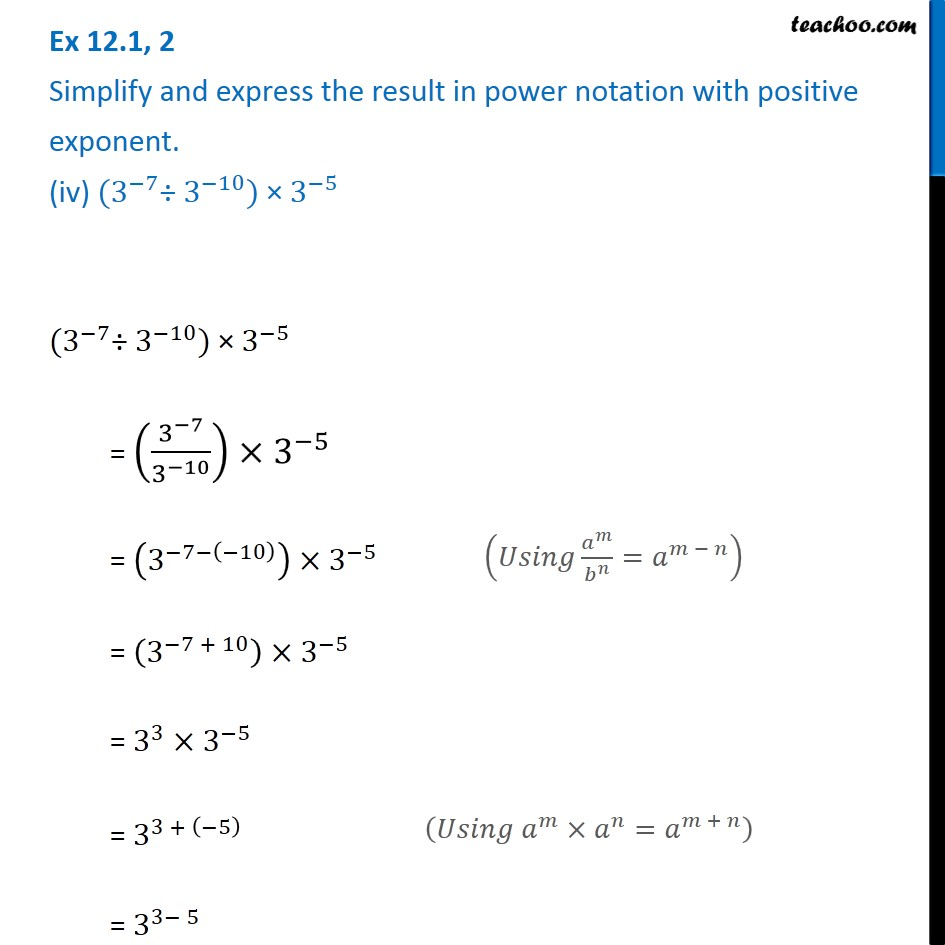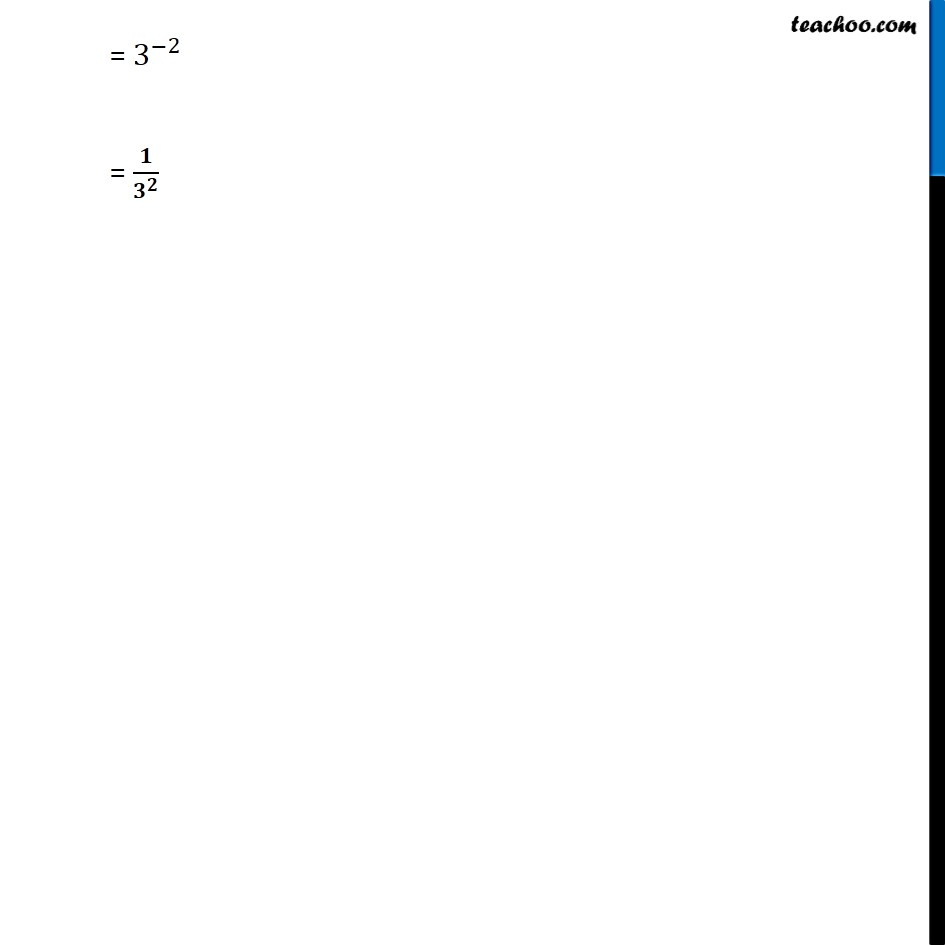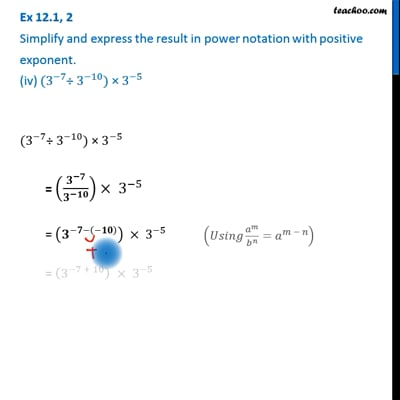Ex 12.1

Chapter 12 Class 8 Exponents and Powers
Serial order wiseThis video is only available for Teachoo black users

Get live Maths 1-on-1 Classs - Class 6 to 12

### Transcript

Ex 12.1, 2 Simplify and express the result in power notation with positive exponent. (iv) 〖(3〗^(−7)÷ 3^(−10)) × 3^(−5) 〖(3〗^(−7)÷ 3^(−10)) × 3^(−5) = (3^(−7)/3^(−10) )×3^(−5) = (3^(−7−(−10) ) )×3^(−5) = (3^(−7 + 10) )×3^(−5) = 3^3×3^(−5) = 3^(3 + (−5) ) = 3^(3− 5 ) (𝑈𝑠𝑖𝑛𝑔 𝑎^𝑚/𝑏^𝑛 =𝑎^(𝑚 − 𝑛) ) (𝑈𝑠𝑖𝑛𝑔 𝑎^𝑚×𝑎^𝑛=𝑎^(𝑚 + 𝑛) ) = 3^(−2) = 𝟏/𝟑^𝟐